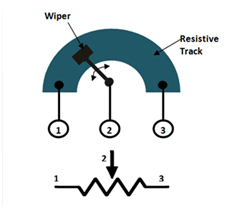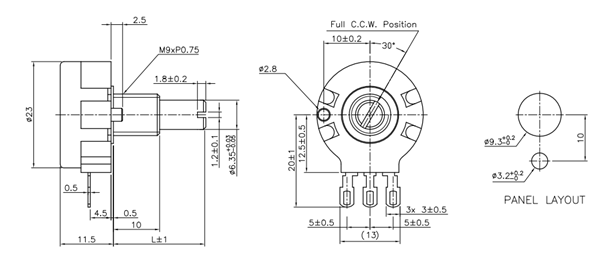# Potentiometer

[Click the image to enlarge it]

### Potentiometer Pin Configuration

 Pin No. Pin Name Description 1 Fixed End This end is connected to one end of the resistive track 2 Variable End This end is connected to the wiper, to provide variable voltage 3 Fixed End This end is connected to another end of the resistive track

### Features

• Type: Rotary a.k.a Radio POT
• Available in different resistance values like 500Ω, 1K, 2K, 5K, 10K, 22K, 47K, 50K, 100K, 220K, 470K, 500K, 1 M.
• Power Rating: 0.3W
• Maximum Input Voltage: 200Vdc
• Rotational Life: 2000K cycles

Note: Complete Technical Details can be found at the datasheet given at the end of this page.

### Alternative Variable Resistor

Resistors, Trimmers, TrimPot

### Selecting a Potentiometer

Potentiometers also known as POT, are nothing but variable resistors. They can provide a variable resistance by simply varying the knob on top of its head. It can be classified based on two main parameters. One is their Resistance (R-ohms) itself and the other is its Power (P-Watts) rating.

The value or resistance decides how much opposition it provides to the flow of current. The greater the resistor value the smaller the current will flow. Some standard values for a potentiometer are 500Ω, 1K, 2K, 5K, 10K, 22K, 47K, 50K, 100K, 220K, 470K, 500K, 1 M.

Resistors are also classified based on how much current it can allow; this is called Power (wattage) rating. The higher the power rating the bigger the resistor gets and it can also more current. For potentiometers the power rating is 0.3W and hence can be used only for low current circuits.

### How to Use a Potentiometer

As far as we know resistors should always have two terminals but, why a potentiometer has three terminals and how to we use these terminals. It is very easy to understand the purpose of these terminals by looking at the diagram below.The diagram shows the parts present inside a potentiometer. We have a resistive track whose complete resistance will be equal to the rated resistance value of the POT.

As the symbol suggests a potentiometer is nothing but a resistor with one variable end. Let us assume a 10k potentiometer, here if we measure the resistance between terminal 1 and terminal 3 we will get a value of 10k because both the terminals are fixed ends of the potentiometer. Now, let us place the wiper exactly at 25% from terminal 1 as shown above and if we measure the resistance between 1 and 2 we will get 25% of 10k which is 2.5K and measuring across terminal 2 and 3 will give a resistance of 7.5K.

So the terminals 1 and 2 or terminals 2 and 3 can be used to obtain the variable resistance and the knob can be used to vary the resistance and set the required value.

### Applications

• Voltage and Current Control Circuits
• Used as volume control knobs in radios
• Tuning or controlling circuits

### 2D Diagram (Model P232)Component Datasheet